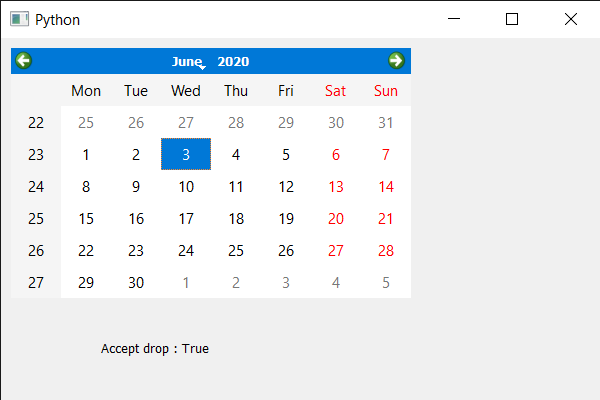GeeksforGeeks App
Open AppBrowser
Continue

# PyQt5 QCalendarWidget – Getting accept drops property

In this article we will see how we can get the accept drop property of the QCalendarWidget. We know we can make drag enabled using setDragEnabled method with calendar object, but what is the use of dragging the text we can’t drop it any where. Allowing accept drop means that calendar has a property now to accept the drop text of the other calendar widget. Accepting drops is used for setting year as it is the spin box object as child, by default this property is false but can be changed with the help of setAcceptDrops method.

In order to do this we will use acceptDrops method with the QCalendarWidget object.

Syntax : calendar.acceptDrops()

Argument : It takes no argument

Action Performed : It return bool

Below is the implementation

## Python3

 `# importing libraries``from` `PyQt5.QtWidgets ``import` `*``from` `PyQt5 ``import` `QtCore, QtGui``from` `PyQt5.QtGui ``import` `*``from` `PyQt5.QtCore ``import` `*``import` `sys`  `class` `Window(QMainWindow):` `    ``def` `__init__(``self``):``        ``super``().__init__()` `        ``# setting title``        ``self``.setWindowTitle(``"Python "``)` `        ``# setting geometry``        ``self``.setGeometry(``100``, ``100``, ``600``, ``400``)` `        ``# calling method``        ``self``.UiComponents()` `        ``# showing all the widgets``        ``self``.show()` `    ``# method for components``    ``def` `UiComponents(``self``):` `        ``# creating a QCalendarWidget object``        ``calendar ``=` `QCalendarWidget(``self``)` `        ``# setting geometry to the calendar``        ``calendar.setGeometry(``10``, ``10``, ``400``, ``250``)` `        ``# accepting drops``        ``calendar.setAcceptDrops(``True``)` `        ``# creating a label``        ``label ``=` `QLabel(``self``)` `        ``# setting geometry to the label``        ``label.setGeometry(``100``, ``280``, ``250``, ``60``)` `        ``# making label multi line``        ``label.setWordWrap(``True``)` `        ``# getting accept drops property``        ``value ``=` `calendar.acceptDrops()` `        ``# setting text to the label``        ``label.setText(``"Accept drop : "` `+` `str``(value))`  `# create pyqt5 app``App ``=` `QApplication(sys.argv)` `# create the instance of our Window``window ``=` `Window()` `# start the app``sys.exit(App.``exec``())`

Output :My Personal Notes arrow_drop_up